# 屌丝把水壶扔了说这是生活常识，用天然气和用电烧开1kg的水，哪个更便宜？

• 生活常识
• 2021-11-11 23:14:02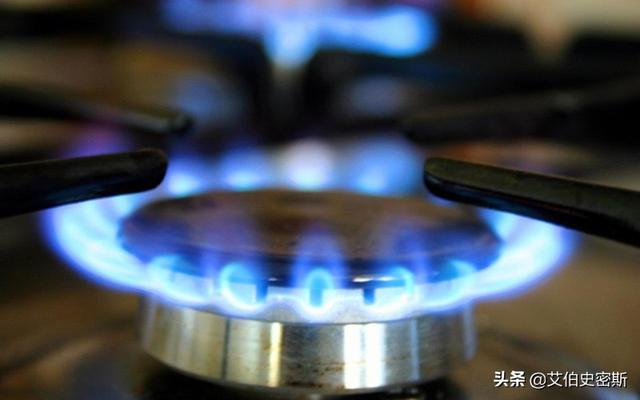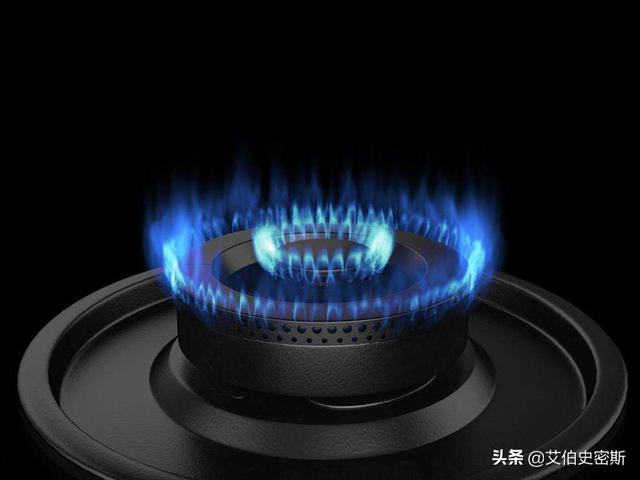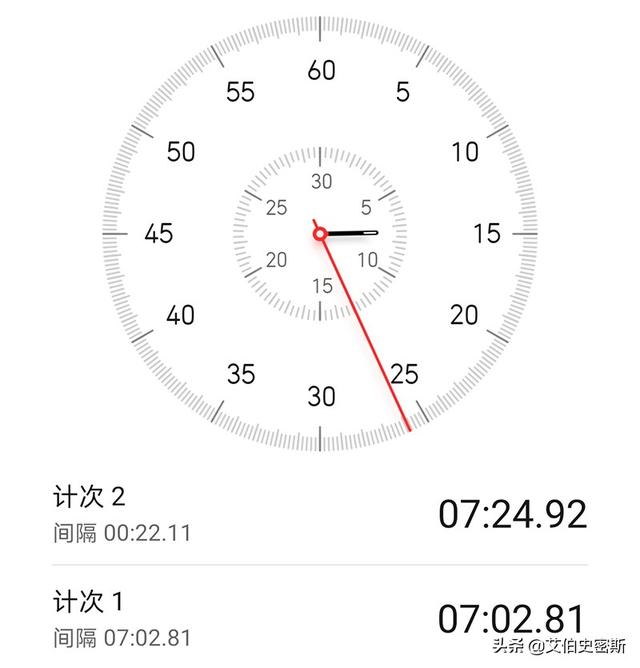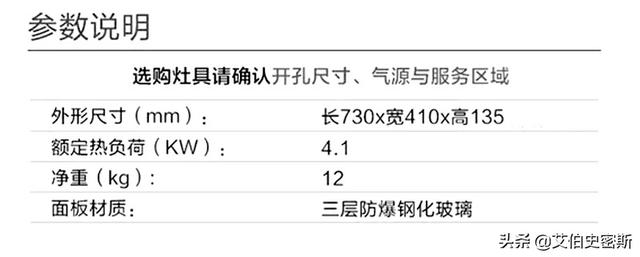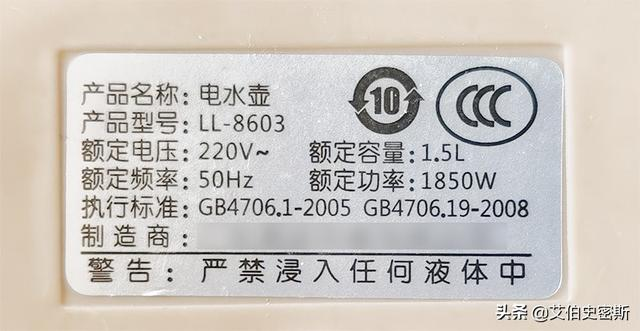Q=1kg*(100℃-20℃)*4200=3.36*10^5焦耳；

（1）燃气灶

V=Q/(60%*3.5*10^7)=0.016立方米；

（2）电热水壶

E=3.36*10^5/0.85=3.95*10^5焦耳=0.11度；

（1）燃气灶

V=423*0.41/3600≈0.048立方米;

（2）电热水壶

E=1850*445=8.2*10^5焦耳=0.23度；

•关注微信